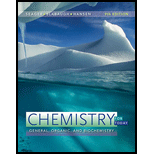Chapter 8, Problem 8.16E### Chemistry for Today: General, Orga...

9th Edition
Spencer L. Seager + 2 others
ISBN: 9781305960060

#### Solutions

Chapter
Section### Chemistry for Today: General, Orga...

9th Edition
Spencer L. Seager + 2 others
ISBN: 9781305960060
Textbook Problem

# A reaction generates chlorine gas ( Cl 2 ) as a product. The reactants are mixed and sealed in a 250 .-mL container. After 30 .0   minutes , 2.97 × 10 − 2   mol of Cl 2 has been generated. Calculate the average rate of the reaction.

Interpretation Introduction

Interpretation:

The average rate of the given reaction on mixing reactants sealed in a 250.-mL container and measuring the number of moles of Cl2 as 2.97×102mol after 30.0minutes is to be calculated.

Concept introduction:

The average rate of a reaction is defined as the rate of change of concentration of products formed or reactant consumed in a particular time interval. It is given by the formula represented below as,

Rate=ΔCΔt

Explanation

The formula to calculate molarity is given below as,

M=molesofsolutelitersofsolution

Substitute the value of number of moles of Cl2 and volume of container in the above equation as follows.

M=molesofCl2volumeofcontainer=2.97×102mol250mL×1L1000mL=0.1188M

The formula to calculate average rate of a reaction is given below as,

Rate=ΔCΔt

Where,

ΔC is the change in concentration

### Still sussing out bartleby?

Check out a sample textbook solution.

See a sample solution

#### The Solution to Your Study Problems

Bartleby provides explanations to thousands of textbook problems written by our experts, many with advanced degrees!

Get Started

## Additional Science Solutions

#### Find more solutions based on key concepts